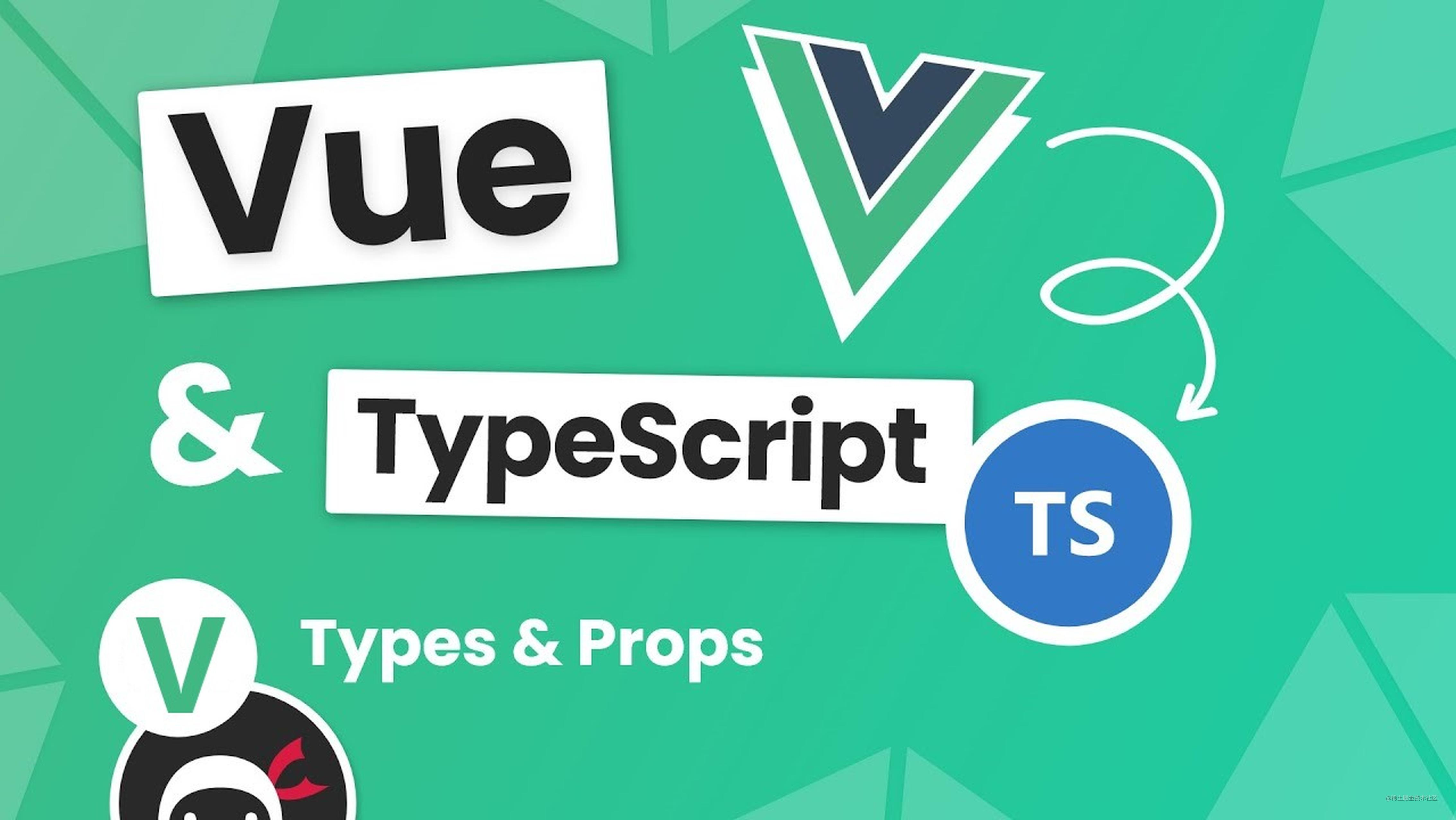# 如何为 Vue3 组件标注 TS 类型，看这个就够了！## 为 props 标注类型

### 使用 <script setup>

<script setup lang="ts">
const props = defineProps({
foo: { type: String, required: true },
bar: Number
})

props.foo // string
props.bar // number | undefined
</script>

<script setup lang="ts">
const props = defineProps<{
foo: string
bar?: number
}>()
</script>

// or
<script setup lang="ts">
interface Props {
foo: string
bar?: number
}

const props = defineProps<Props>()
</script>

interface Props {
msg?: string
labels?: string[]
}

const props = withDefaults(defineProps<Props>(), {
msg: 'hello',
labels: () => ['one', 'two']
})

### 非 <script setup>

import { defineComponent } from 'vue'

export default defineComponent({
props: {
message: String
},
setup(props) {
props.message // <-- 类型：string
}
})

## 为 emits 标注类型

### 使用 <script setup>

<script setup> 中，emit 函数的类型标注也可以使用 运行时声明 或者 基于类型的声明

<script setup lang="ts">
// 运行时
const emit = defineEmits(['change', 'update'])

// 基于类型
const emit = defineEmits<{
(e: 'change', id: number): void
(e: 'update', value: string): void
}>()
</script>

### 非 <script setup>

import { defineComponent } from 'vue'

export default defineComponent({
emits: ['change'],
setup(props, { emit }) {
emit('change') // <-- 类型检查 / 自动补全
}
})

## 为 ref() 标注类型

### 默认推导类型

ref 会根据初始化时的值自动推导其类型：

import { ref } from 'vue'

// 推导出的类型：Ref<number>
const year = ref(2020)

// => TS Error: Type 'string' is not assignable to type 'number'.
year.value = '2020'

### 通过接口指定类型

import { ref } from 'vue'
import type { Ref } from 'vue'

const year: Ref<string | number> = ref('2020')

year.value = 2020 // 成功！

### 通过泛型指定类型

// 得到的类型：Ref<string | number>
const year = ref<string | number>('2020')

year.value = 2020 // 成功！

// 推导得到的类型：Ref<number | undefined>
const n = ref<number>()

## 为 reactive() 标注类型

### 默认推导类型

reactive() 也会隐式地从它的参数中推导类型：

import { reactive } from 'vue'

// 推导得到的类型：{ title: string }
const book = reactive({ title: 'Vue 3 指引' })

### 通过接口指定类型

import { reactive } from 'vue'

interface Book {
title: string
year?: number
}

const book: Book = reactive({ title: 'Vue 3 指引' })

## 为 computed() 标注类型

### 默认推导类型

computed() 会自动从其计算函数的返回值上推导出类型：

import { ref, computed } from 'vue'

const count = ref(0)

// 推导得到的类型：ComputedRef<number>
const double = computed(() => count.value * 2)

// => TS Error: Property 'split' does not exist on type 'number'
const result = double.value.split('')

### 通过泛型指定类型

const double = computed<number>(() => {
// 若返回值不是 number 类型则会报错
})

## 为事件处理函数标注类型

<script setup lang="ts">
function handleChange(event) {
// event 隐式地标注为 any 类型
console.log(event.target.value)
}
</script>

<template>
<input type="text" @change="handleChange" />
</template>

function handleChange(event: Event) {
console.log((event.target as HTMLInputElement).value)
}

## 为 provide / inject 标注类型

provide 和 inject 通常会在不同的组件中运行。要正确地为注入的值标记类型，Vue 提供了一个 InjectionKey 接口，它是一个继承自 Symbol 的泛型类型，可以用来在提供者和消费者之间同步注入值的类型：

import { provide, inject } from 'vue'
import type { InjectionKey } from 'vue'

const key = Symbol() as InjectionKey<string>

provide(key, 'foo') // 若提供的是非字符串值会导致错误

const foo = inject(key) // foo 的类型：string | undefined

const foo = inject<string>('key') // 类型：string | undefined

const foo = inject<string>('foo', 'bar') // 类型：string

const foo = inject('foo') as string

## 为 dom 模板引用标注类型

<script setup lang="ts">
import { ref, onMounted } from 'vue'

const el = ref<HTMLInputElement | null>(null)

onMounted(() => {
el.value?.focus()
})
</script>

<template>
<input ref="el" />
</template>

## 为组件模板引用标注类型

<!-- MyModal.vue -->
<script setup lang="ts">
import { ref } from 'vue'

const isContentShown = ref(false)
const open = () => (isContentShown.value = true)

defineExpose({
open
})
</script>

<!-- App.vue -->
<script setup lang="ts">
import MyModal from './MyModal.vue'

const modal = ref<InstanceType<typeof MyModal> | null>(null)

const openModal = () => {
modal.value?.open()
}
</script>

Ok，以上就是在 Vue3 组件中使用 TS 类型的基本方法，也是我最近的 Vue3 学习笔记。欢迎在评论区交流讨论，一起学习成长！